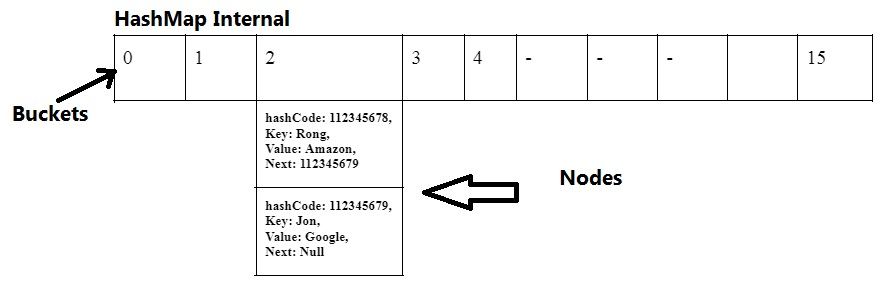Tutorials Hut

• # Internal Working Of Java HashMap

Internal Working Of Java HashMap is based on bucketing and hashing principle. Let’s look into all necessary concepts to understand hashmap internal working.

## What is Hashing

Hashing is a technique where we try to generate smaller unique values for given input and the returned value will always be unique for given unique input, for the same input it will always return the same output. Mostly for any input hashing algorithm generates a unique int number.

## What is Bucketing

Whenever we use hashing, it generates a big integer number as hashCode and if we use such numbers, it will be hard to manage such big numbers, so we pass the hashCode to another functions to give a smaller number, usually the number that it returns from such functions are called bucket number. Bucket numbers are always defined for example 0-15 and so on. They are usually smaller numbers.

## What is initialCapacity And loadFactor in Java Collection

initialCapacity – initailCapacity is a parameter which is usually passed as contractor argument while creating a collection, it initializes the collection with that capacity. For example if we create a map with initialCapacity of 16 then it will have the ability to store 16 elements at start.

loaFactor – It is a parameter which is usually passed as a constructor argument while creating a collection, it is used to determine when the collection is about to get full and then increases the capacity of collection by doubling it.

## Example of InitialCapacity and LoadFactor:

Let’s say we have 16 initial capacity and load factor 0.75 in a collection, so the threshold value will be 16*0.75 = 12, and as soon as the 12th element (threshold) will be added, it will double the capacity of collection.

Initialization of HashMap and Storing a Value in Map(Working of put(key, value) method)
Whenever we create a HashMap without any specific initialCapacity and loadFacotor, it creates a hashMap with initial capacity of 16 and load factor 0.75. When we add an element in hashMap it generates hashCode for the key value of element then passes this to another function to find bucket number, initially bucket number will be between 0-15 (Remember capacity is 16 initially). As soon as the bucket number is determined it adds the element with hashCode, key and value to that bucket.

If we add another element the same process gets repeated. What will happen if two buckets are the same for two elements (key, value) ? In that case we will add a new element to the end of the previous element. Each element in the bucket is a kind of linkedList where elements of the linked list are always pointing to the previous and next element.

## How to Find a Value In Hash Map (Working of get(key) method)

When we call get() method with key value, it goes back to the same algorithm to find the hashCode for the key and then the bucket number. Once we have a bucket number, we get to that bucket, we check for key and hashCode if matches we can return the value or move to the next element in the same bucket until we find the correct value.

## Example of Internal Working Of HashMap

See below diagram where 0-15 total (16) slots are representing initial capacity and buckets of hashMap. Assume we want to add a key value pair (“Rong”, “Amazon”) where “Rong” is key and “Amazon” is value.

The key “Rong” will be passed to hashing algorithm to find a int hashCode, assume hashCode is 112345678, this hashCode will be passed to find bucket number, let’s say bucket number is 2 (Remember our initial bucket numbers are 0-15 in hashMap and capacity is 16). So it will go to that bucket number and store hashCode, key value pair (“Rong”, “Amazon”).

Similarly if we add another element (“Jon”, “Google”) and assume the bucket number is same then it will add this new element next to the previous one.Now let’s say we want to find the value for key “Jon”, then same process will repeat where we will find hashCode (112345679) for “Jon” and then we will find bucket number (2) and got to that bucket and match hashCode, key and if no match we will move to the next until we find it or reach to the null.